Скачать презентацию Digital Signal Processing Prof Nizamettin AYDIN naydin yildiz edu

6baac61d99cfe63486b458827f33999b.ppt

• Количество слайдов: 29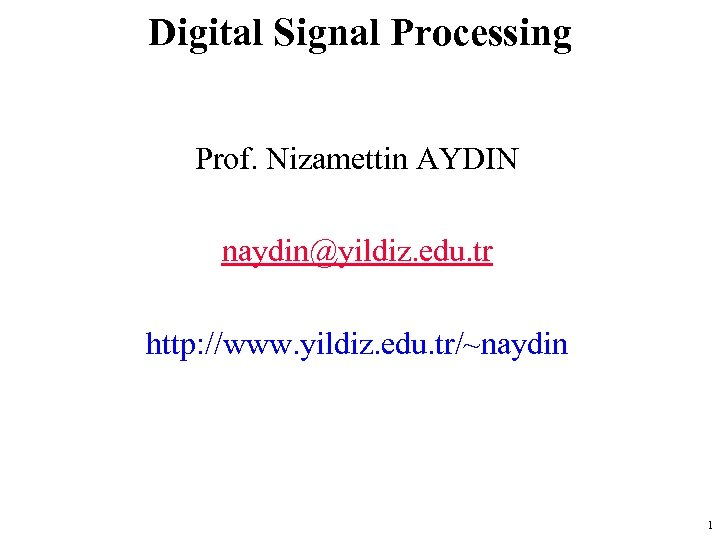Digital Signal Processing Prof. Nizamettin AYDIN naydin@yildiz. edu. tr http: //www. yildiz. edu. tr/~naydin 1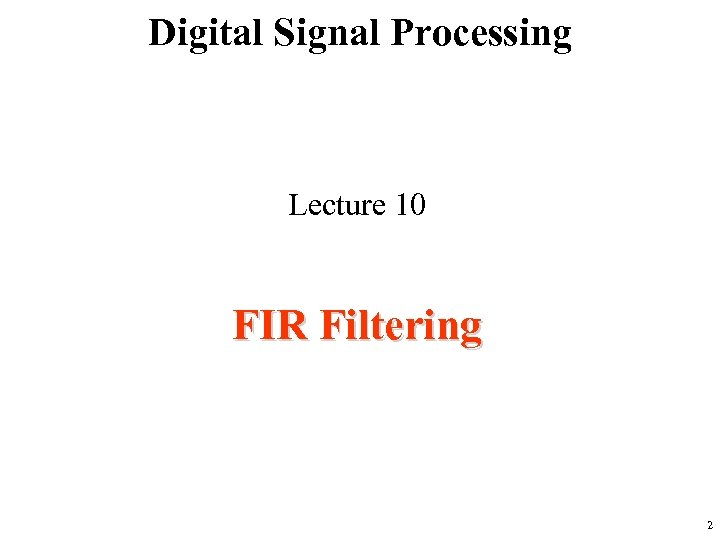Digital Signal Processing Lecture 10 FIR Filtering 2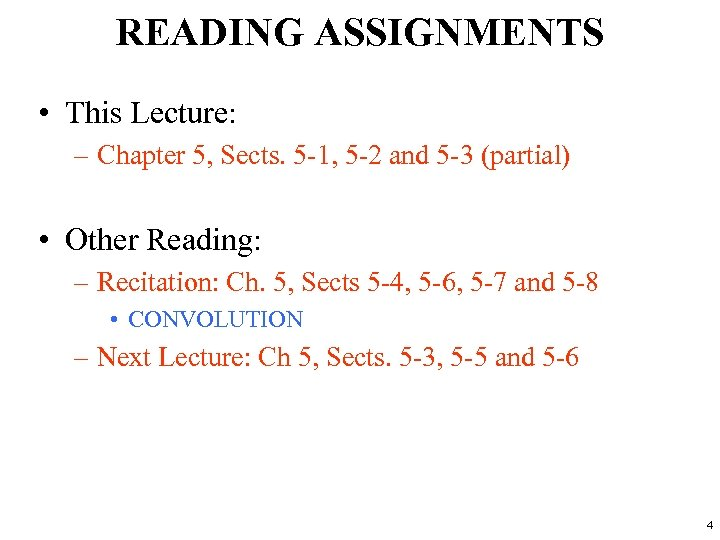READING ASSIGNMENTS • This Lecture: – Chapter 5, Sects. 5 -1, 5 -2 and 5 -3 (partial) • Other Reading: – Recitation: Ch. 5, Sects 5 -4, 5 -6, 5 -7 and 5 -8 • CONVOLUTION – Next Lecture: Ch 5, Sects. 5 -3, 5 -5 and 5 -6 4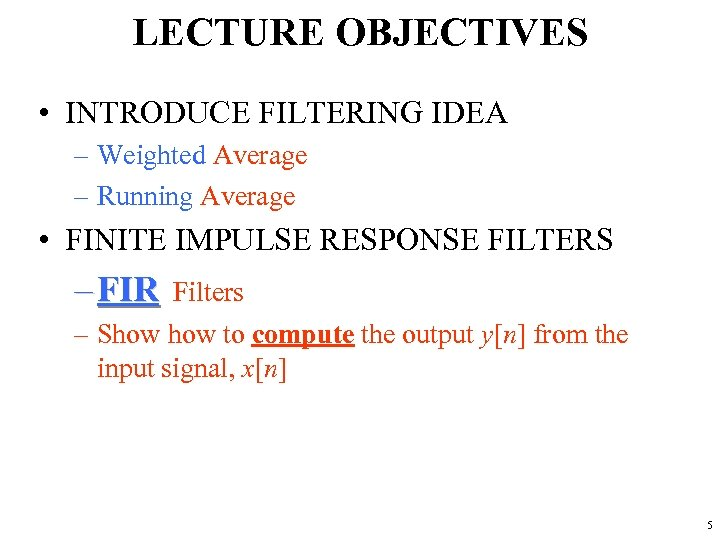LECTURE OBJECTIVES • INTRODUCE FILTERING IDEA – Weighted Average – Running Average • FINITE IMPULSE RESPONSE FILTERS – FIR Filters – Show to compute the output y[n] from the input signal, x[n] 5DIGITAL FILTERING x(t) A-to-D x[n] COMPUTER y[n] D-to-A y(t) • CONCENTRATE on the COMPUTER – PROCESSING ALGORITHMS – SOFTWARE (MATLAB) – HARDWARE: DSP chips, VLSI • DSP: DIGITAL SIGNAL PROCESSING 6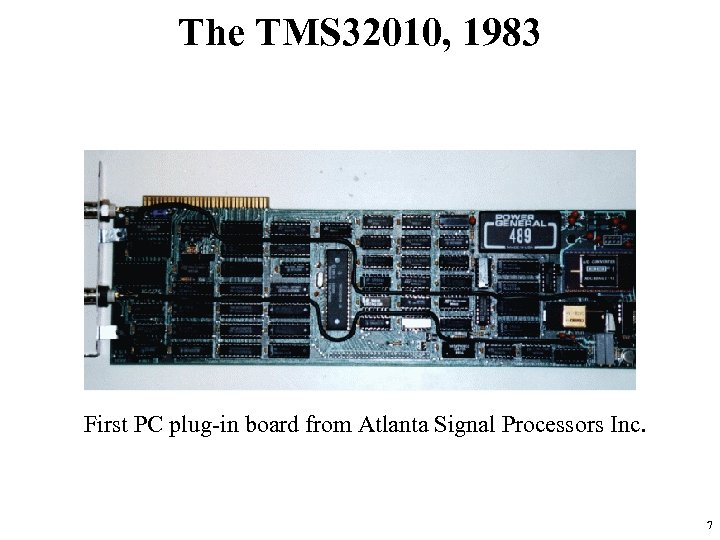The TMS 32010, 1983 First PC plug-in board from Atlanta Signal Processors Inc. 7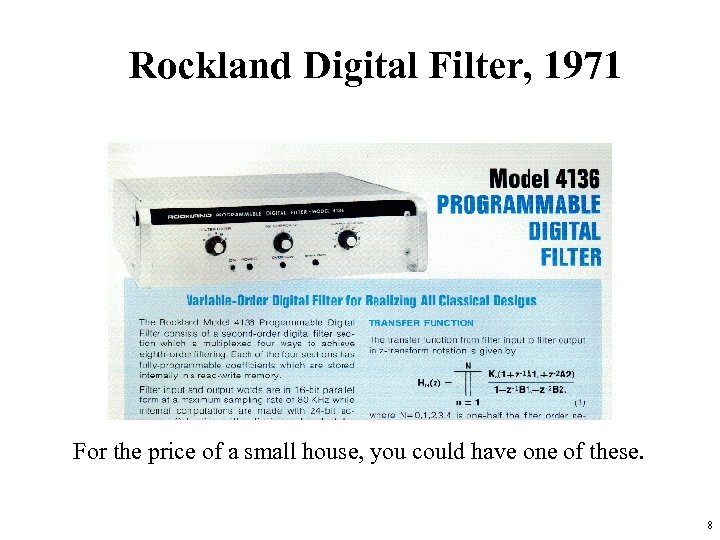Rockland Digital Filter, 1971 For the price of a small house, you could have one of these. 8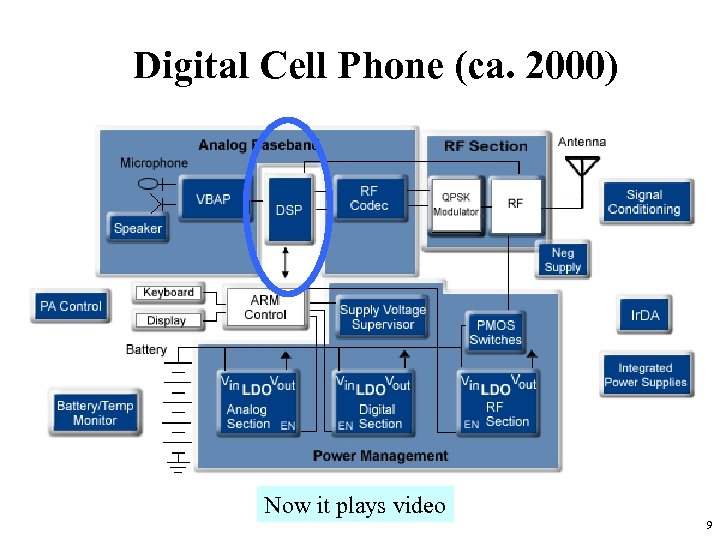Digital Cell Phone (ca. 2000) Now it plays video 9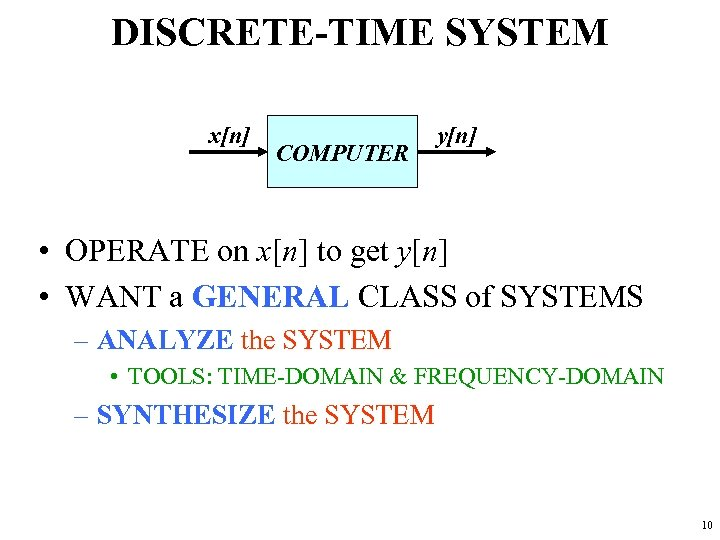DISCRETE-TIME SYSTEM x[n] COMPUTER y[n] • OPERATE on x[n] to get y[n] • WANT a GENERAL CLASS of SYSTEMS – ANALYZE the SYSTEM • TOOLS: TIME-DOMAIN & FREQUENCY-DOMAIN – SYNTHESIZE the SYSTEM 10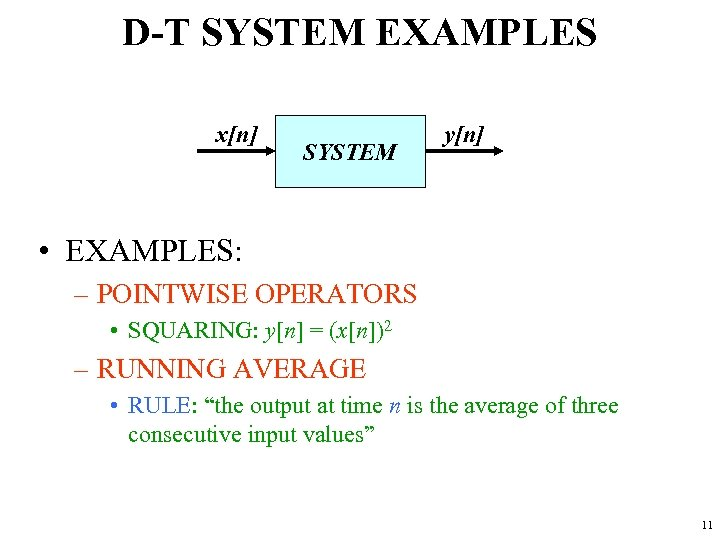D-T SYSTEM EXAMPLES x[n] SYSTEM y[n] • EXAMPLES: – POINTWISE OPERATORS • SQUARING: y[n] = (x[n])2 – RUNNING AVERAGE • RULE: “the output at time n is the average of three consecutive input values” 11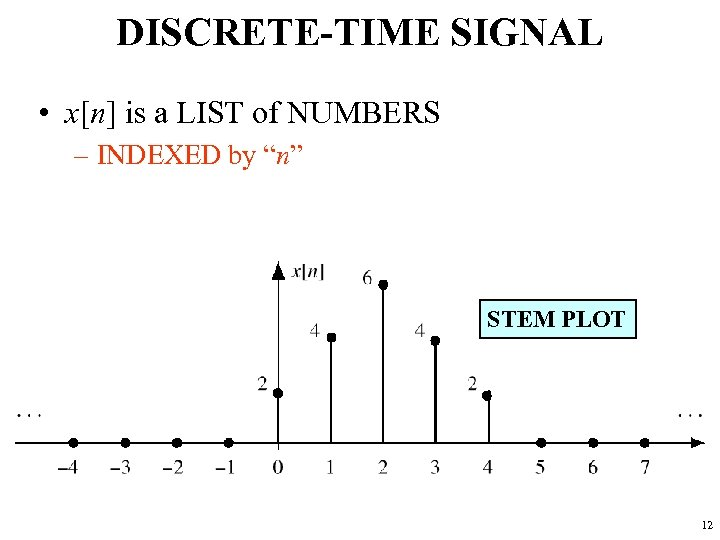DISCRETE-TIME SIGNAL • x[n] is a LIST of NUMBERS – INDEXED by “n” STEM PLOT 12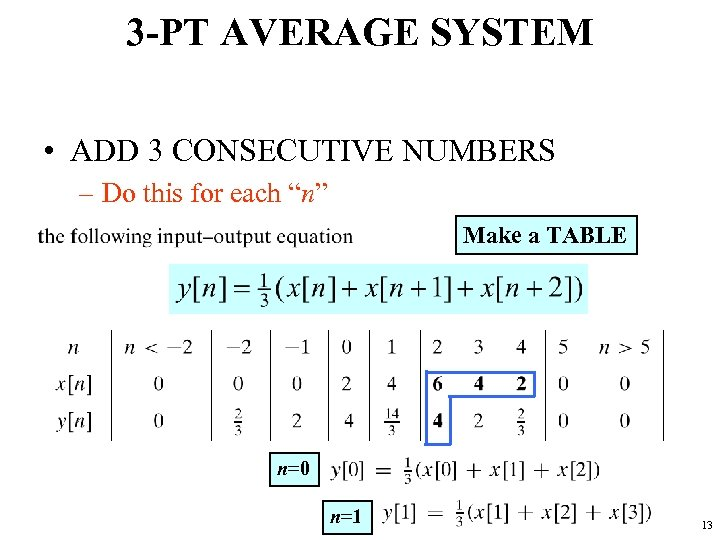3 -PT AVERAGE SYSTEM • ADD 3 CONSECUTIVE NUMBERS – Do this for each “n” Make a TABLE n=0 n=1 13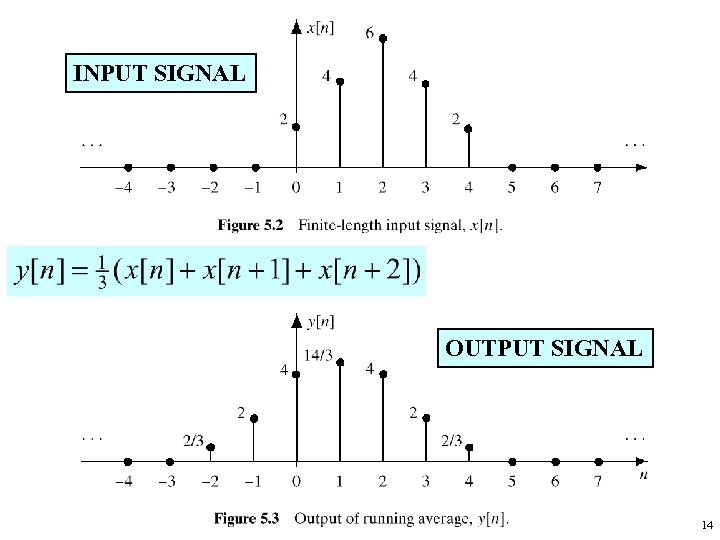INPUT SIGNAL OUTPUT SIGNAL 14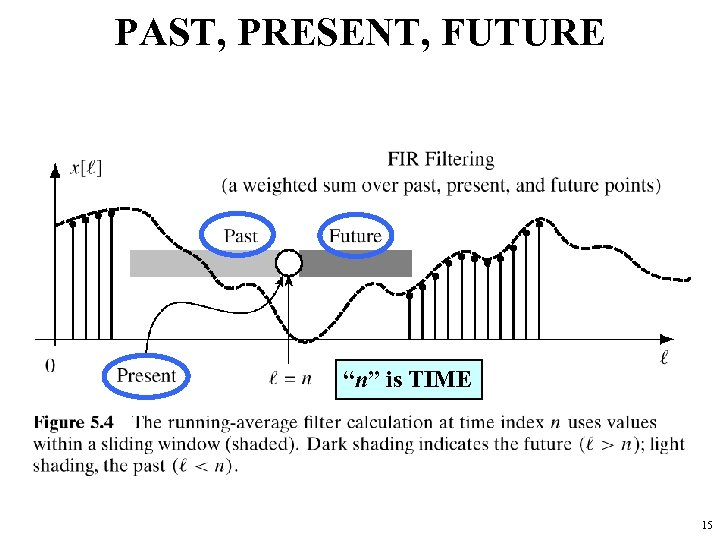PAST, PRESENT, FUTURE “n” is TIME 15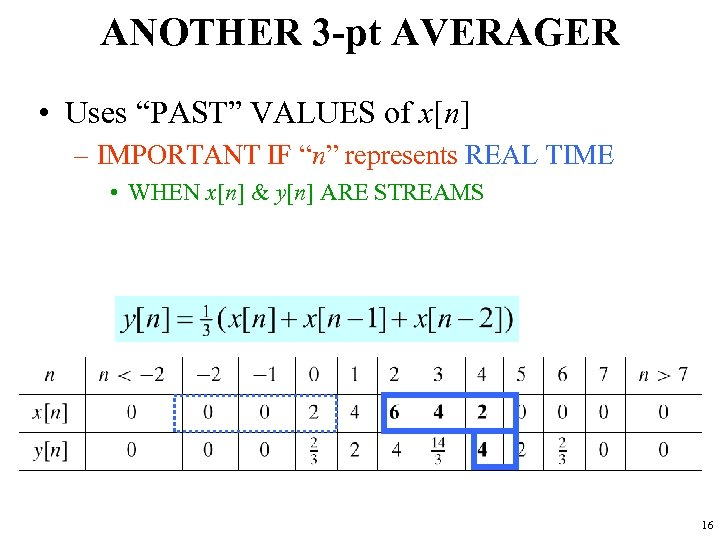ANOTHER 3 -pt AVERAGER • Uses “PAST” VALUES of x[n] – IMPORTANT IF “n” represents REAL TIME • WHEN x[n] & y[n] ARE STREAMS 16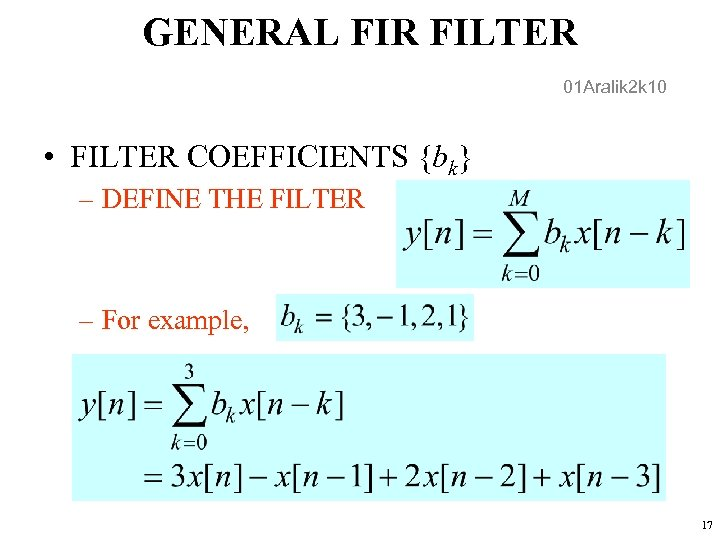GENERAL FIR FILTER 01 Aralik 2 k 10 • FILTER COEFFICIENTS {bk} – DEFINE THE FILTER – For example, 17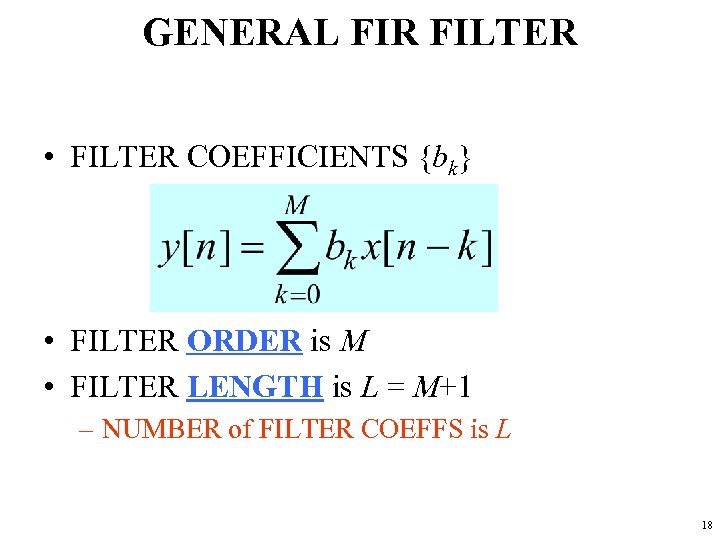GENERAL FIR FILTER • FILTER COEFFICIENTS {bk} • FILTER ORDER is M • FILTER LENGTH is L = M+1 – NUMBER of FILTER COEFFS is L 18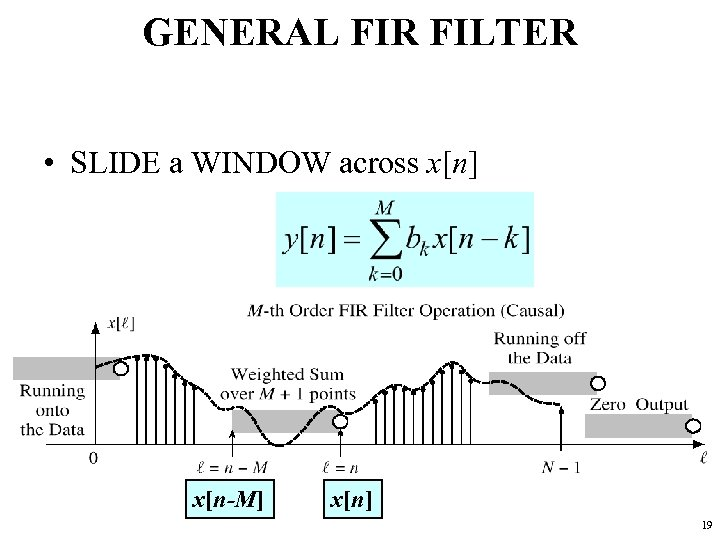GENERAL FIR FILTER • SLIDE a WINDOW across x[n] x[n-M] x[n] 19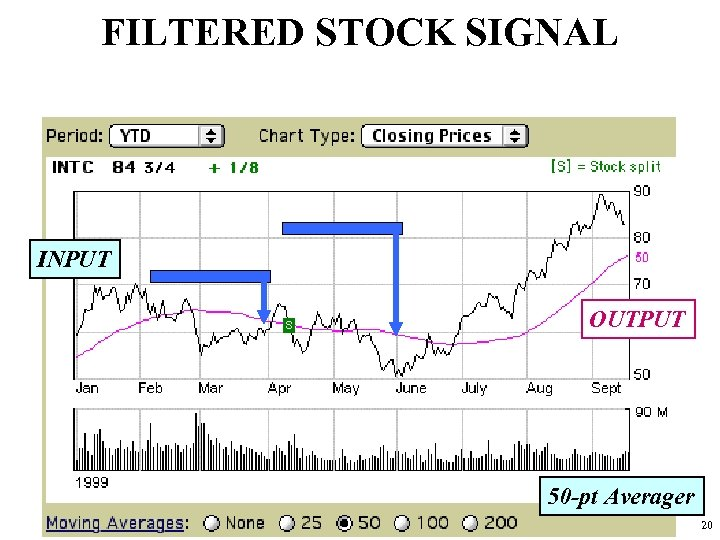FILTERED STOCK SIGNAL INPUT OUTPUT 50 -pt Averager 20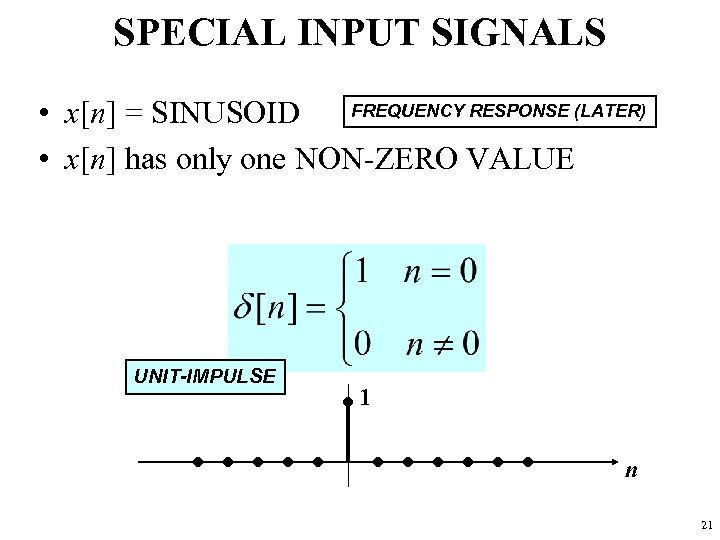SPECIAL INPUT SIGNALS FREQUENCY RESPONSE (LATER) • x[n] = SINUSOID • x[n] has only one NON-ZERO VALUE UNIT-IMPULSE 1 n 21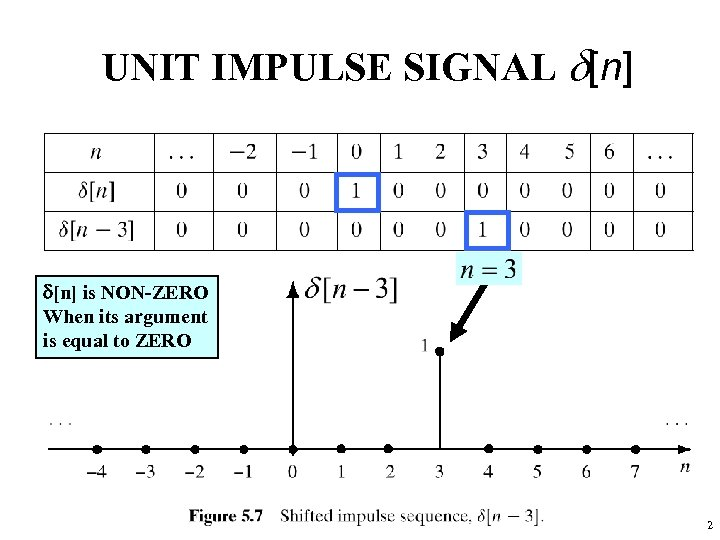UNIT IMPULSE SIGNAL d[n] is NON-ZERO When its argument is equal to ZERO 22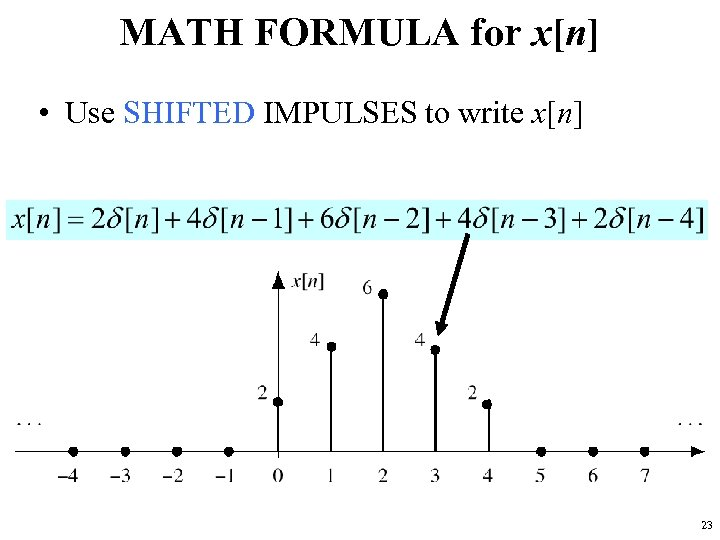MATH FORMULA for x[n] • Use SHIFTED IMPULSES to write x[n] 23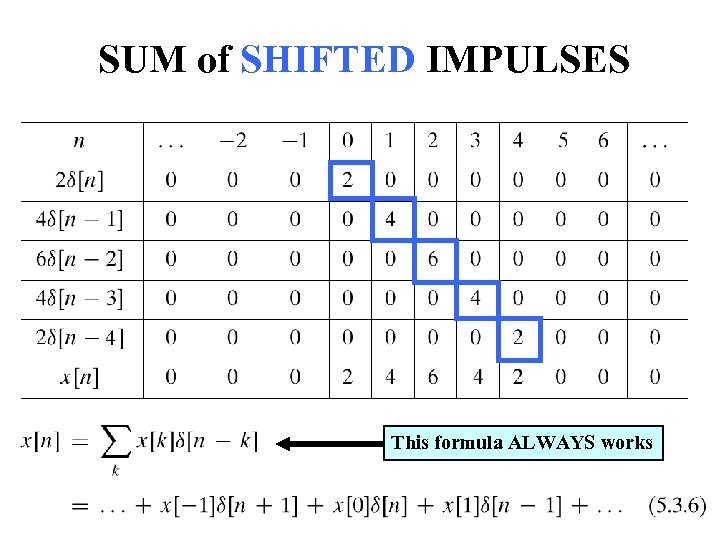SUM of SHIFTED IMPULSES This formula ALWAYS works 24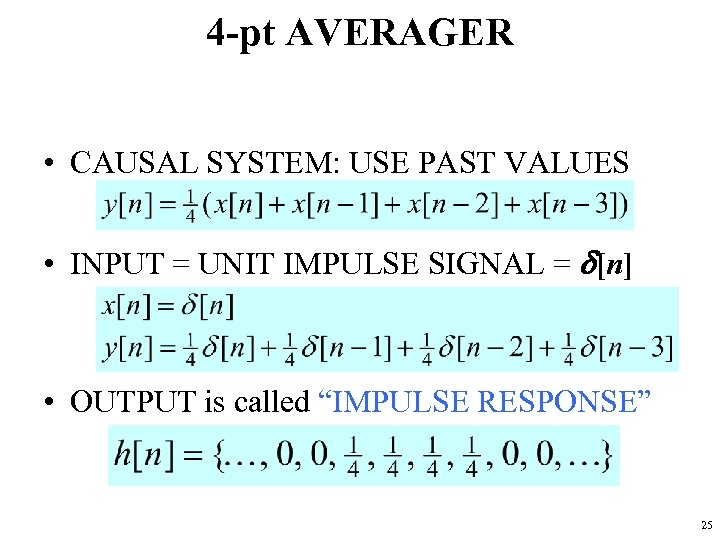4 -pt AVERAGER • CAUSAL SYSTEM: USE PAST VALUES • INPUT = UNIT IMPULSE SIGNAL = d[n] • OUTPUT is called “IMPULSE RESPONSE” 25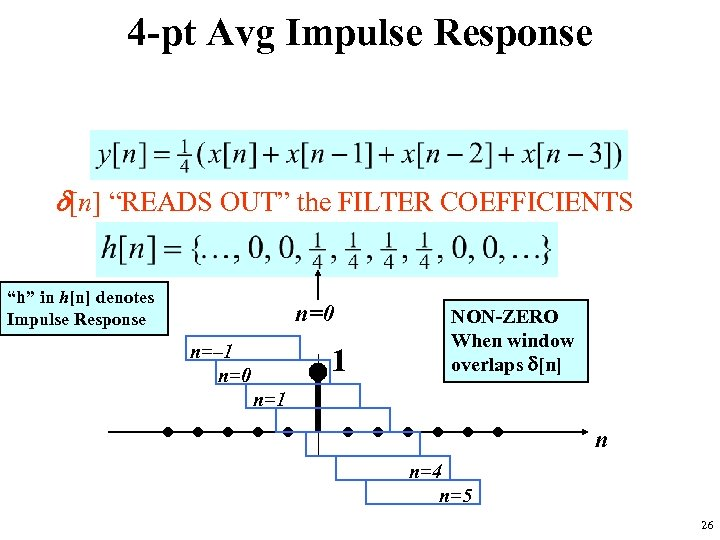4 -pt Avg Impulse Response d[n] “READS OUT” the FILTER COEFFICIENTS “h” in h[n] denotes Impulse Response n=0 n=– 1 n=0 1 NON-ZERO When window overlaps d[n] n=1 n n=4 n=5 26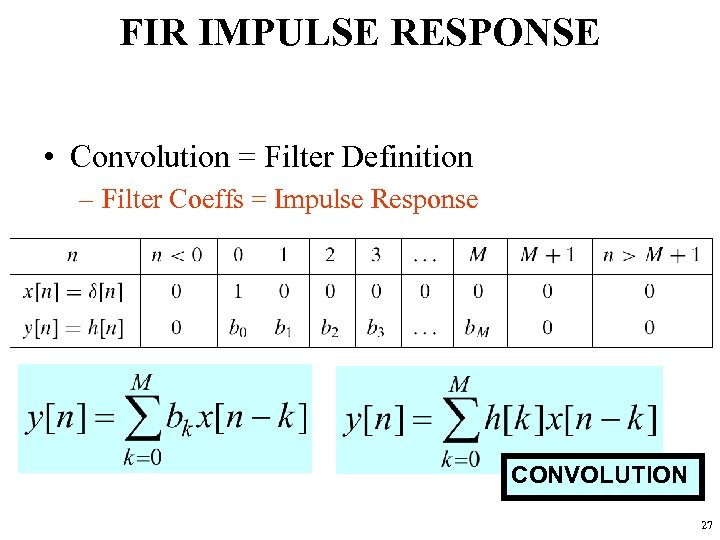FIR IMPULSE RESPONSE • Convolution = Filter Definition – Filter Coeffs = Impulse Response CONVOLUTION 27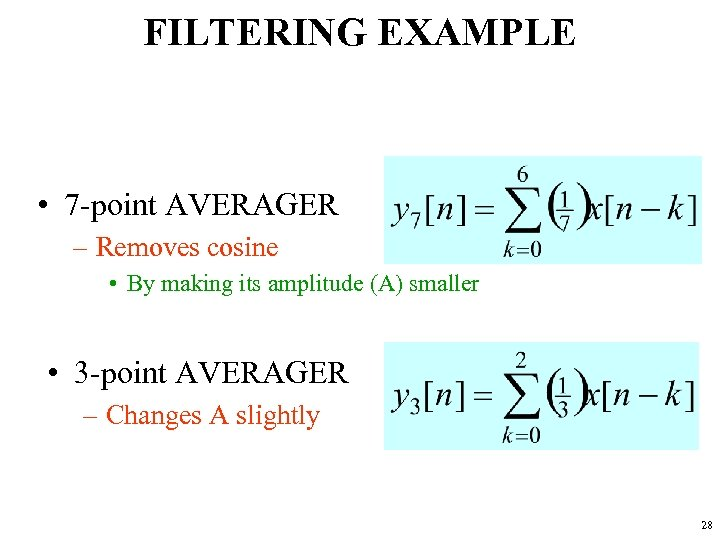FILTERING EXAMPLE • 7 -point AVERAGER – Removes cosine • By making its amplitude (A) smaller • 3 -point AVERAGER – Changes A slightly 28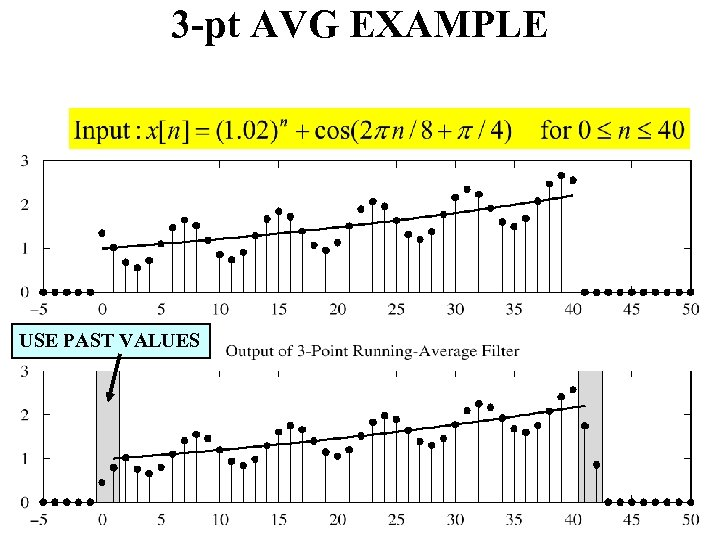3 -pt AVG EXAMPLE USE PAST VALUES 29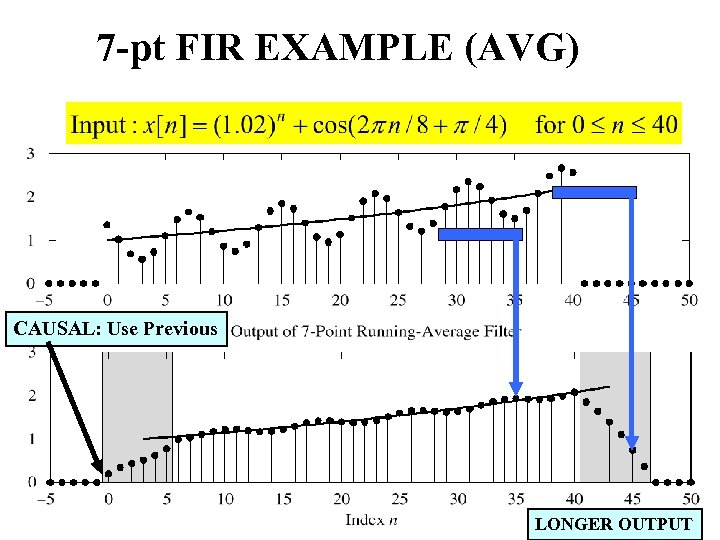7 -pt FIR EXAMPLE (AVG) CAUSAL: Use Previous LONGER OUTPUT 30